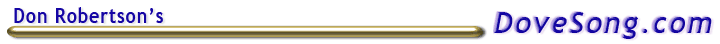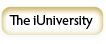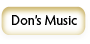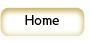DoveSong.Com

### The DoveSongArchives

The Text LibraryPositive Music
Papers/Articles
Movement (2004)Through the Centuries
Overview
Gregorian Chant
15th Century
16th Century
17th Century
18th Century
19th Century
20th Century
21st CenturyGospel Music
Black Gospel
Mountain Gospel
Southern GospelWorld Music
Chinese Music
Indian Music
Persian MusicPopular Music

The MP3 Library
(no longer operational)Western Classical
Plainsong (Chant)
Renaissance
Baroque
RomanticGospel Music
Mountain Gospel
Black Gospel
Southern GospelWorld Music
India
China
Middle East
PersiaPop/Folk/Country/Jazz

Musical Numerology
By Don Robertson, 1970 with revisions in 2003
Copyright 1970, 2003 by Don Robertson
(First appeared in the book Kosmon)

I wrote the article Musical Numerology in 1970 and published it in Denver, Colorado in a book called Kosmon, a book created by myself and two other people. It was in this book that I first published my findings about the duochord and proclaimed that rock and roll music was taking a downward turn.

One day, after we had started distributing the book to local bookstores, I received a letter from a young man who lived nearby and wanted to meet the author of this article. I went to see him and looking around the one-bedroom apartment, I saw that everywhere there were large pieces of white paper on which he had been making mathematical calculations: on tables, chairs, and taped to all of the walls. He was ecstatic, "I had to meet the author because I have applied what was stated in the article to every known science and found that the same formula proves itself in every case." I went back a week later to discuss the matter with him more thoroughly , but the apartment was empty and he was gone.
Now after 23 years, I have revised the article, and am publishing it again on DoveSong.Com. I hope this information can be as useful to others as it was for this young man and has been for me. I believe that musical scales and harmony are based on alchemical realities that can be proved by math. Math demonstrates the formation of the laws of the universe, and music, color, and all manifestation follow the same laws.

Don Robertson, May, 2003

* * * * * * * * * * * * * * * * * * * * * * * * * * * * * * * * *

To our knowledge, there is no religion on the earth that does not incorporate some kind of symbolic use of the quantities three and seven. Our objective is to illustrate some of the mysterious relationships between the quantity three, the quantity seven, and what these quantities have in common with the basic physical structure of the universe.

The direct relationship between three and seven is as follows: If we have three objects, which we will call 1, 2, and 3, we will observe that the absolute number of different combinations of these 3 objects is 7; that is, we may arrange them in no more than seven different combinations, regardless of the order in which they are arranged.

 Combination 1 1, 2, 3 Combination 2 1, 2 Combination 3 1, 3 Combination 4 2, 3 Combination 5 1 Combination 6 2 Combination 7 3

There are three primary colors in the color spectrum, plus four more natural colors that are created from the combinations of the three colors similarly to the three objects above. All colors that exist are either: a) One of the primary colors, or b) A resultant mixture of two or three of the primary colors. The primary colors cannot be created by mixing other colors, so they are rather special. The three primary colors are red, yellow, and blue. The natural, or secondary colors are those colors that are created by mixing equally the three primary colors according to the chart above. The resultant seven natural colors the colors of the rainbow, a naturally occurring phenomena that can be created using a prism to break down light. They are arranged in the spectrum as follows:

## The Color Spectrum

 Red 1 Red Orange 1, 2 Red, Yellow Yellow 2 Yellow Green 2, 3 Yellow, Blue Blue 3 Blue Indio 1, 2, 3 Red, Yellow, Blue Violet 1, 3 Red, Blue

Thus we see that the color spectrum is based on the relationship between 3 and 7.

There are three primary tones of the musical scale and seven natural tones:

## The Musical Scale

 C 1 C D 1, 2 C, E E 2 E F 2, 3 E, G G 3 G A 1, 2, 3 C, E, G B 1, 3 C, G

The notes that correspond to the primary colors are C, E, G: the major triad, which is the most important chord in music, and the groundwork of the scale. It is important to realize the correspondence between the colors of the spectrum and the notes of the scale, as the musical scale is based on the same principals of nature (the natural overtone series) and it has been adapted in some form or another most all cultures.

There are three root-position triads contained in the major scale (C,D,E,F,G,A,B). These are: The major triad that is based on the correspondence with the primary colors (C, E, G) and two minor triads, based on three of the natural, or secondary, tones (D, F, A and E,G,B). Major and minor triads are the fundamental underpinnings of harmony used in the music of Western cultures.

### Three Forces at the heart of Nature

The simplest physical structure in the material universe is the hydrogen atom. It has the least number of forces at work: three. These three forces are:

1)  At the center of the atom resides the nucleus, composed of a positive charge of electricity called a proton.

2)  Orbiting around this proton in an elliptical orbit is a negative charge of electricity called an electron. Since this electron has a negative charge, and since a negative charge is attracted to a positive charge, the electron is attracted to the proton.

3)  Since the electron is attracted to the proton, there needs to be a force that causes equilibrium between the two, as the electron would collide with the proton if no such force were in place. This force then is the force that governs bodies in motion, causing them to travel in an orbit. It is centrifugal force. In the atom, this is the neutron.

### Some Aspects of Three

If there is a given object that is fixed in space, this object has, as its location, three coordinates. An example of this would be the relative position of an airplane above the earth. It is calculated by longitude, latitude, and altitude, its three coordinates.

If someone looks into the corner of a room, where the room meets the walls, they will see three intersecting planes that are considered to extend into infinity. These are the three dimensions of space.

There are three time zones that man senses, past, present, and future.

If there is space with no motion, then time does not exist, only space. But if there is no motion, then there can be no space, for there has to be space to contain the motion. The motion through space causes sequentially. The earth’s orbit around the sun and revolution on its axis causes a time sequence on earth. Thus we have three aspects: time, space, and motion.

The Law of Three

The fact that events, laws, and all creation result from three principals is referred to as the "Law of Three" or the "Law of the Triangle." This law is illustrated by the example that every effect has two causes: one passive, and one active.

 What does the Russian philosopher Georges Gurdieff have to say about the Law of Three? In the book In Search of the Miraculous, PD Ouspensky quotes him as follows:  “This is the ‘Law of Three’ or the law of the three principles or the three forces. It consists of the fact that every phenomenon, on whatever scale and in whatever world it may take place, from molecular to cosmic phenomena, is the result of the combination or the meeting of three different and opposing forces. Contemporary thought realizes the existence of two forces and the necessity of these two forces for the production of a phenomenon: force and resistance, positive and negative magnetism, positive and negative electricity, amel and femail cells, and so on. But it does not observe even these two forces always and everywhere. No question has ever been raised as to the third, or if it has been raised it has scarcely been heard. “According to real, exact knowledge, once force, or two forces, can never produce a phenomenon. The presence of a third force is necessary, for it is only with the help of a third force that the first two can produce what may be called a phenomenon, no matter in what sphere. “The teaching of the three forces is at the root of all ancient systems. The first force may be called active or positive, the second, passive or negative; the third, neutralizing. But these are merely names, for in reality all three forces are equally active and appear as active, passive and neutralizing, only at their meeting points, that is to say, only in relation to one another at a given moment. The first two forces are more or less comprehensible to man and the third may sometimes be discovered either at the point of application of the forces, or in the medium, or in the result. But speaking in general, the third forces is not easily accessible to direct observation and understanding. The reason for this is to be found in the functional limitations of man’s ordinary psychological actively and in the fundamental categories of our perception of the phenomenal world, that is, in our sensations of space and time resulting from these limitations. People cannot perceive and observe the third force directly any more than they can spatially perceive the forth dimension. “The first fundamental law of the universe is the law of three forces, or three principles, or as it is often called, the law of three. According to this law, every action, every phenomenon in all worlds without exception, is the result of a simultaneous action of three forces: the positive, the negative, and the neutralizing. Of this we have already spoken…. The next fundamental law of the universe is the law of seven or the law of octaves.

### Some Aspects of Seven

During the late 1700’s, some chemists began identifying certain chemical elements. About 1800, they began to determine the atomic weights of some of them. In 1808, John Dalton suggested that atoms were physical objects with specific weights. In 1829, Johann Doebereiner showed that particular elements could be arranged in groups of three. In each group, the elements had similar properties and the weight of the middle atom of the group was close to the average of the other two. These were said to be chemical equivalents to the musical triad.

### The Law of Octaves

In 1864, John A. Newlands grouped all the known elements in the order of their atomic weights. He then divided them into groups of seven elements each. He showed that when the atoms were put into order with their weights increasing, there was a repetition in the similarities according to the musical octave, the same found on the keyboard. When he talked to chemists of this time about his newly found law of octaves, they laughed.

But octaves are the substance of the musical scale, the same musical scale that was demonstrated by Pythagoras and used today, with slight modifications according to culture. It is a naturally occurring phenomena. The basis of the musical scale is the octave, the repetition of the vibration of the set of seven notes that occurs as the fundamental vibration of the first note, the basic note of that scale, is doubled.

In 1869, Mendeleev compiled a “Periodic Table of the Elements.” This table was arranged according to atomic weights. Mendeleev found, as had Newlands, that the chemical properties of the elements reoccur at definite intervals. He concluded that these were the periodic functions of their atomic weights. According to Daniel Morris, in his article Music of the New Spheres in the December, 1969 Chemistry Magazine, Mendeleev’s arrangement of the elements came to him while listening to a performance of Schumann’s exquisite piano quintet. He was seated upon a sofa, apparently mulling over Newland’s and Doebereiner’s arrangements of the elements, when all of a sudden he jumped up, sat at his desk, and arranged all of the elements accordng to a new plan. Morris' feeling was that there was a definite relationship between the quintet and the periodic table in the repetition and development of the seven-note melody of the quintet.

### Vibrations in Different Octaves

It was in 1925 that French scientist Louis Victor Pierre Raymond duc de Broglie discovered that the electron was actually a wave of vibration. These waves properly follow the same property and characteristics of vibration as the vibrating string of a musical instrument, the waves being complete with overtones. (We are familiar with the presence of overtones on the vibrating string from the experiments of Pythagoras and his monochord – a single-stringed instrument he built to display how musical scales were produced by the natural overtone series).

An atom such as uranium has more that 90 electrons, each capable of its own harmonies, and all this can be recorded by a spectroscope. From this, one can conclude that all matter is made of waves. Therefore, we, and the world we live in, are vibrations…just as the children of the 1960s discovered while taking large doses of LSD! But this should not be too surprising. Our understanding of the world around us is through perception of the five senses. What we experience is actually taking place in our brain, interpretations of waves of light, taste, smell, feeling, and sound. Are we really living in a world, as we suspect, or is this simply a world created in our brain, similar to that when we dream? And if spectral and aural vibrations obey the same law of octaves that applies to the physical makeup of the universe, then perhaps it is all just a part of one large keyboard of vibrations, with particular sensations and manifestations taking place in particular octave ranges. Light, heat, magnetic and chemical vibration are all subject to the law of octaves.

The ancient Greek philosopher Democritus wrote:

Sweet and bitter
Cold and warm,
As well as all the colors.
All these things exist
But in the opinion
And not in reality.

What really exists
Are unchangeable articles: atoms,
And their motions in empty space.

The great German mathematician Gottfried Wilhelm Leibniz wrote: “I am able to prove that not only light, color, heat, and the like, but motion, shape and extension too are mere apparent qualities.” Many scientists and philosophers have come to the conclusion that everything exists in the mind: stars, planets, atoms. Einstein showed us that even space and time were forms of intuition.

Georges Gurdjieff, the important Russian spiritual teacher said that the law of octaves was based upon the discontinuity of all vibrations in nature, as they do not develop uniformly, but with periodic accelerations and retardations. The original impulse becomes alternately stronger and weaker. This could be a phenomenon similar to the AC cycle. This cycle of alternating electrical vibrations can be viewed by means of an oscilloscope or with a computer program such as Sound Forge. Called the duty cycle, it appears as such.

####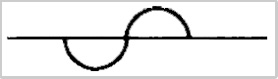All sounds are made up of these cycles. The cycles take place in the time domain. By using a brilliant mathematical formula known as the Fourier transform, these cycles can be translated into the frequency domain, where we find that the timber of the sound depends upon the strength of overtones, the higher harmonics of the fundamental tone.

The duty cycle can be equated to a circle broken at its diameter with the bottom half twisted 180 degrees or to the continuous movement of a spiral. Alternating current (AC) obeys the laws of the duty cycle: energy alternates with no energy. Home electricity in the United States alternates sixty times per second, thus it is called 60 cycles, or 60 Hertz. An electric light bulb does not continually burn, but flashes sixty times in one second, faster than our eyes can follow, so we perceive the appearance only of continuous light. This would not be the case when light is powered by a battery, as batteries produce direct current (DC).

Musical tones can be represented using the mathematical symbols of trigonometry, signs and cosigns, and the imaginary numbers of calculus. Computer systems that generate musical tones, such as synthesizers, do so using these mathematical formulas. All of this is based on the alternation of the duty cycle.

### “Shocks”

While teaching in Russia during the first world war, Gurdjieff described something about octaves that had not been publically known before. He stated that there were two places in the octave where retardation of the vibrations takes place. These places are between the notes E and F and between the notes B and C. These are the two places on the keyboard where there are no sharp or flat note (black) keys between the white keys. Octaves, according to Gurdjieff, develop according to whether or not these places where retardation of vibrations take place are “filled.” In the right development of these octaves, these places are filled by the energy of octaves that run in parallel to the given octave. This process of filling in Gurdjieff calls a shock. Processes, institutions, laws and other things all progress through time according to the law of octaves, and therefore these two shocks in the octave are a part of the development  process. Gurdjieff stated that if development continues without the shocks being filled in, then the line of development constantly changes. The way he described it, if octaves – as a principal of nature – develop unimpeded, the development looks as if it is proceeding along a straight line, but every time one of the two points in the octave is reached where a shock occurs, even though development of the process that the octave represents appears to be progressing forward along a straight line, the development of the process actually jogs a little, causing what would be compared to a jog in the line. The development continues and after the progress through the series of octaves has gone on for a particular amount of time, what was considered to be development along a straight line has actually turned into a development in the opposite direction! Further continuation will bring the development back to the same direction that it started in. This is actually a full duty cycle, or one full revolution on a spiral.

The law of octaves, with this intrinsic circular motion generating a spiral, governs all activity and shows how organizations, religions, and governments -- if allowed to develop without conscious knowledge concerning the application of the ‘filling in’ of these shocks -- always develop into something that is opposite to what was originally intended.

### The Octave with Two Added Shocks

Gurdjieff explained how an octave of seven ‘notes’ was transformed into one of nine, the seven notes and the two shocks, by applying the following fascinating bit of arithmetic.

He divided the number ‘1’ into seven equal fractions:

1/7, 2/7, 3/7, 4/7, 5/7, 6/7, 7/7

The decimal equivalents of these seven fractions are arrived at using the time-honored tradition of dividing the numerators by the denominator:

1/7 = .142857
2/7 = .285714
3/7 = .428571
4/7 = .571428
5/7 = .714285
6/7 = .857142

Of course 7/7 equals 1

Studying the numbers that result from our division is a fascinating process. We find that we have a reoccurring sequence of numbers that is present in every case! This is the sequence 7142857142857….that continues on and on ad infinitum.

.142857142857142857 etc….
7/1.00000000000000000

(Numerical patterns such as these are very telling. They describe a situation that is naturally occurring and should be taken seriously by the researcher).

The nature of this pattern is very interesting. It starts with the number ‘7’, which is the number of the octave, the number that we are dealing with here. Next in line is double that number, ‘14’. Following that we have another doubling: ‘28’, and after that another doubling: ‘56’…whoops, not 56, but 57, the ‘7’ being the start of another sequence! It takes some thought about this to fully appreciate the beauty of this sequence and this change from 56 to 57 to start another series. This just really breathes “cycle,” does it not?

There are other interesting things about this series of numbers. If we add each line in the set of six decimals above, we always get the number ‘28’ as a result. This is because each of the six decimal sequences is ordered in such a way that when you add downwards, only the six numbers of the sequence appear, there are no duplications. We have six pieces of the whole (7/7), each with a sequence of six numbers, the same sequence in each piece, ordered in the very unique fashion. This is something to contemplate.

Now we will turn to something called horizontal addition. This is a process used in numerology. Although we don’t endorse the common understanding of what numerology represents, the principles behind manipulation of numbers are simple aspects of natural mathematics, which demonstrate the laws of nature, and this understanding of numbers and what they are all about is the key to understanding nature and what it is all about. That is why trigonometry and calculus are so important to engineering. Both these sciences give us an understanding of nature, and hence, an understanding of sound, and the natural laws that govern the use of sound in music…principals that are not arbitrary, but work according to natural law, the law of three, the law of octaves, and now the law of nine (the octave plus the two shocks).

By using horizontal addition on the sequence, we arrive at another number:

1 + 4 + 2 + 8 + 5 + 7 = 27

2 + 7 = 9

Adding the sequence numbers together, we end up with ‘9’. This demonstrates one way that seven ties into nine.

The Periodicity of Nine

The notes of the octave combined with the two shocks show us what is called the periodicity of nine. Creating a circle with nine positions and connecting the numbers from our sequence above, we have the figure that Gurdjieff calls the enneagram.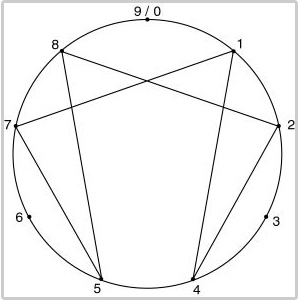The numbers that are not in the sequence are 3, 6, and 9. When a they are connected, the result is a triangle. The numbers 3 and 6 are the shocks.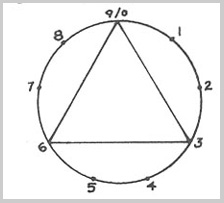By applying the color spectrum and musical notes to the enneagram, we have the following: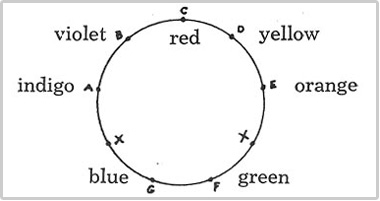When asked why one shock correctly appeared between the E and the F, but the other, instead of appearing between B and C, appeared between G and A, Gurdjieff stated that this demonstrated the nature of the shock that occurred when passing from one octave to the next. He also said that every completed whole, every organism, is an enneagram, but that each of these enneagrams does not have an inner triangle (3,6,9). 142857 is the working of the corporeal scheme, the triangle is the presence of higher elements in the organism. This inner triangle is possessed by plants, for example, from which come our “drugs”: hemp, poppy, hops, tea, coffee, tobacco.

When any number is added to nine, the sum, added horizontally to one significant figure, always equals that same number (also added horizontally to one significant figure).

Examples:

8 + 9 = 17  and  1 + 7 = 8

6 + 9 = 15  and   1 + 5 = 6

36 + 9 = 45  and  4 + 5 = 9  and 3 + 6 = 9

When any number is multiplied by nine, the product added horizontally to one significant figure, always equals nine.

Examples:

9 x 7 = 63  and 6 + 3 = 9

9 x 12 = 108 and 1 + 0 + 8 = 9

Casting Out Nines

Further use of horizontal addition is applied in a mathematical process that is called casting out nines. The sum of any number added horizontally is equal to the remainder of that same number when it is divided by nine. Thus, to cast out all nines from a number, add it horizontally:

Example:

16,378 = 1 + 6 + 3 + 7 + 8 = 25 = 7

If you were to divide 16,378 by 9, you will find that the remainder is 7.

The Enneagram with Combinations of Nine

This interesting drawing shows how multiplication of nine by the other single-digit numbers produces a sum that fits nicely into our enneagram. We have equated nine with zero because nine becomes zero when it is cast. Notice how the sums are equal to the two numbers at that level of the drawing (for example, the sums on the first line are 18 and 81, on the second 72 and 27 and so on).We see that by horizontal addition of the sums, in each case, we always end up with nine. If we now take each number from one to eight and multiply it by numbers 1 through 9 – we will see some interesting numerical patterns develop. We will start with the first line from the circle above:

8 x 1 = 8
8 x 2 = 7 (16, or 1 + 6 = 7)
8 x 3 = 6 (24, or 2 + 4 = 6, and so on…)
8 x 4 = 5
8 x 5 = 4
8 x 6 = 3
8 x 7 = 2
8 x 8 = 1
8 x 9 = 9

We end up now with a sequence of 876543219. A similar sequence is produced by doing the same thing with 1. This sequence is the reverse of the other:

1 x 1 = 1
1 x 2 = 2
1 x 3 = 3
1 x 4 = 4
1 x 5 = 5
1 x 6 = 6
1 x 7 = 7
1 x 8 = 8
1 x 9 = 9

This is how this sequence looks on the circle: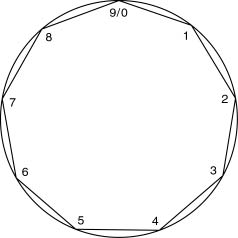Notice that this sequence was generated by the numbers 8 and 1, the two numbers at the top of the enneagram. The number series that resulted from these two was the same, however one series is the reverse of the other. For 8 we have: 876543219, and for 1 we have 12345789. To be completely the opposite of each other, the second series would have had to have been 987654321, but interestingly enough, this isn't the case.

Now we will generate another pair of sequences using seven and two, the next set of numbers in the enneagram.

 7 x 1 = 7 7 x 2 = 5 7 x 3 = 3 7 x 4 = 1 7 x 5 = 8 7 x 6 = 6 7 x 7 = 4 7 x 8 = 2 7 x 9 = 9 2 x 1 = 2 2 x 2 = 4 2 x 3 = 6 2 x 4 = 8 2 x 5 = 1 2 x 6 = 3 2 x 7 = 5 2 x 8 = 7 2 x 9 = 9

This is how this sequence looks on the circle: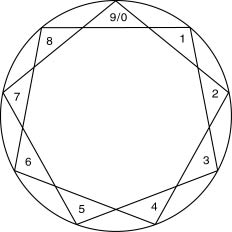Again we have a resultant series of numbers where one is the opposite of the other. For 7 we have 753186429, and for 2 we have 246813579. As is the case with 8 and 1 above, both series always ends with a 9! Also, the reverse series is one number off from the original. This series of numbers is one that skips every other number.

Now we will generate another pair of sequences using five and four:

 5 x 1 = 5 5 x 2 = 1 5 x 3 = 6 5 x 4 = 2 5 x 5 = 7 5 x 6 = 3 5 x 7 = 8 5 x 8 = 4 5 x 9 = 9 4 x 1 = 4 4 x 2 = 8 4 x 3 = 3 4 x 4 = 7 4 x 5 = 2 4 x 6 = 6 4 x 7 = 1 4 x 8 = 5 4 x 9 = 9

This is how this sequence looks on the circle: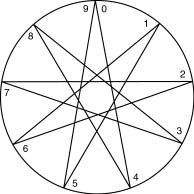When we look at these, we see again that we have the same kind of pattern where the series from the second set is the opposite of the first, however offset by a single number, and that both end in 9. The series for 5 is 516273849 and the series for 4 is 483726159. This series of numbers is one where three numbers is skipped each time

Finally we will take a look at the sequence generated by the two shock values three and six:

 6 x 1 = 6 6 x 2 = 3 6 x 3 = 9 6 x 4 = 6 6 x 5 = 3 6 x 6 = 9 6 x 7 = 6 6 x 8 = 3 6 x 9 = 9 3 x 1 = 3 3 x 2 = 6 3 x 3 = 9 3 x 4 = 3 3 x 5 = 6 3 x 6 = 9 3 x 7 = 3 3 x 8 = 6 3 x 9 = 9

This is how this sequence looks: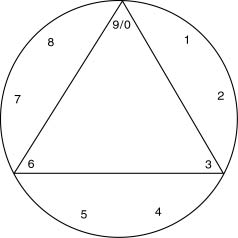Interestingly enough, the number 6 yields a series of 639, 639, 639, and the series from 3 yields the opposite: 369, 369, 369. Who says the universe isn't the result of mathematics!

We have now horizontally multiplied each number from 1 to 9 by the numbers 1 through 9 and have arrived at a series that is in each case has been illustrated using the enneagram circle, the circle with 9 points. The numbers 1,2,4,5,7, and 9 are the numbers found in the period (142857). When we look at the tables that we have just created using these numbers (that is the tables for "8 and 1", "7 and 2", and "5 and 4") we can see that a series of numbers has been created in each table. For example, in the 8 table, the results from horizontally multiplying 1 through 9 by 8 resulted in a pattern (reading down the sum column) of 876543219 and the results from horizontally multiplying 7 by 1 through 9 is the sequence 753186429. Now, taking the third, sixth, and ninth numbers in each of these series, we discover that we have a repeating sequence of either 639 or 369. These are the "inner triangles" of each one of these sets

 8 x 1 = 8 8 x 2 = 7 8 x 3 = 6 8 x 4 = 5 8 x 5 = 4 8 x 6 = 3 8 x 7 = 2 8 x 8 = 1 8 x 9 = 9 1 x 1 = 1 1 x 2 = 2 1 x 3 = 3 1 x 4 = 4 1 x 5 = 5 1 x 6 = 6 1 x 7 = 7 1 x 8 = 8 1 x 9 = 9 7 x 1 = 7 7 x 2 = 5 7 x 3 = 3 7 x 4 = 1 7 x 5 = 8 7 x 6 = 6 7 x 7 = 4 7 x 8 = 2 7 x 9 = 9 2 x 1 = 2 2 x 2 = 4 2 x 3 = 6 2 x 4 = 8 2 x 5 = 1 2 x 6 = 3 2 x 7 = 5 2 x 8 = 7 2 x 9 = 9 5 x 1 = 5 5 x 2 = 1 5 x 3 = 6 5 x 4 = 2 5 x 5 = 7 5 x 6 = 3 5 x 7 = 8 5 x 8 = 4 5 x 9 = 9 4 x 1 = 4 4 x 2 = 8 4 x 3 = 3 4 x 4 = 7 4 x 5 = 2 4 x 6 = 6 4 x 7 = 1 4 x 8 = 5 4 x 9 = 9 order:639 order:369 order:369 order:639 order:639 order:369

We will now show each number and the order of its series. This order, as you have just seen, was determined by the inner triangle of each series (the points of 3, 6 and 9 on the circle) and number present at those point turn out to be either a sequence of 639 or 369. 639 represents energy moving counter clockwise and 369 is the energy moving clockwise. For purposes of demonstration, we will fix polarity designations to the 639 and 369. We will call 369 positive and 639 negative. The numbers 3,6 and 9, not being part of the periods, we will call neutral (=):

 Note Number Order Polarity C 0 = D 1 369 + E 2 639 - (shock) 3 = F 4 369 + G 5 639 - (shock) 6 = A 7 369 + B 8 639 - C 9 =

We will turning again to the sequences that we created above. For simplicity sake, we will just use the forward sequence from each set. These sequences were: 123456789 (from 8-1), 135792468 (from 7-2) 639639639 (from 6-3) and 159483726 (from 5-4). Applying the polarities we assigned in the chart above to these sequences we get:

 8-1 1 2 3 4 5 6 7 8 9 + - = + - = + - = 7-2 1 3 5 7 9 2 4 6 8 + = - + = - + = - 6-3 6 3 9 6 3 9 6 3 9 (all neutral) 5-4 1 5 9 4 8 3 7 2 6 + - = + - = + - =

 It is noted that modern theory of colors has adapted new colors to represent the subtractive domain of primary colors, and some writers go so far as to dispute the standard primary colors, but we stand by what we have written: red, yellow and blue are the primary colors. For more information about modern color theories, see: http://www.goatrance.de/mahak/colors.html http://www.physicsclassroom.com/Class/light/U12L2d.html